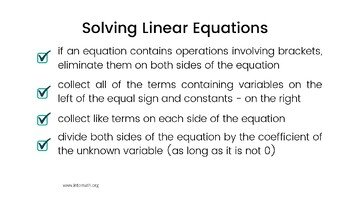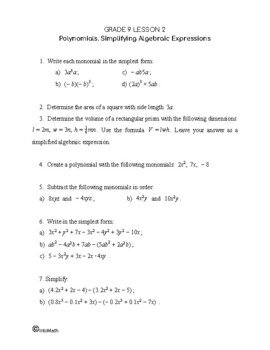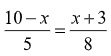CA$2.00 In this lesson you will learn what a linear equation is and what it means to “solve an equation”. In order to solve an equation, we need to complete inverse operations in the reverse BEDMAS order. The solution or root is the value that we get when we solve an equation. This lesson explains the steps of solving linear equations with brackets. From simplifying the expressions with brackets on both sides of the equal sign; identifying, rearranging and collecting like terms to isolating for the unknown variable by dividing by its coefficient.Grade 9 Workbook (14 pages with answers) CA$4.00

In this lesson you will see a concrete example of how to solve an equation that contains brackets, does not initially look like a linear equation, but simplifies to it.

Practice exercises will help you master the skills required for solving linear equations with brackets and multi-step equations.

### Solving linear equations short animation

#### Grade 9 Solving Equations Quiz

Solve the following equation:Solve the following equation: